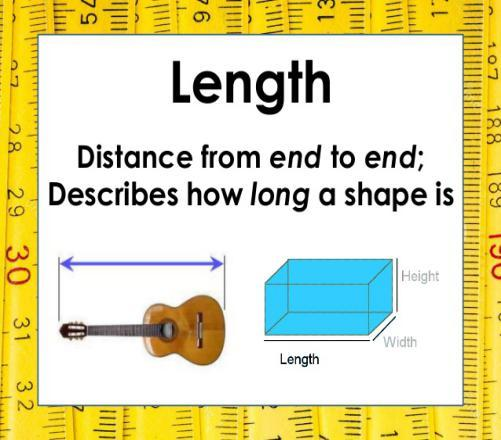# What Do You Know About Length And Size?

10 Questions | Total Attempts: 154SettingsAll objects or entity in the universe has certain propertied to them and unique to certain objects such as their length and size, how about we learn about what comprises of length and size itself. Take a quiz and see what you know about length and size.

• 1.
The measuring of magnitude or dimension of an object or how big something is such as its length, height, diameter, perimeter, mass or areas is referred to as?
• A.

Perimeter

• B.

Shape

• C.

Length

• D.

Size

• 2.
Size is determined by the process of comparing or measuring objects, which results in the determination of the magnitude of a quantity such as?
• A.

Top and bottom

• B.

• C.

Area or volume

• D.

Length or mass

• 3.
Sometimes, an object magnitude is usually expressed as a numerical value of units on a previously established spatial scale such as?
• A.

Inches

• B.

Size

• C.

Scale

• D.

None of the above

• 4.
One common means of perceiving size is to compare the size of a newly observed object with the size of a familiar object whose size is already known, this comparison is done by human using?
• A.

Visual cues

• B.

Subjective vision

• C.

Binocular

• D.

None of the above

• 5.
Human have the capacity for depth perception, which can be used to judge which of several objects is closer, and by how much, which allows for some estimation of the size of the more distant relative object to the closer one, this vision is termed?
• A.

Serendipity

• B.

Visual cues

• C.

Bipolar

• D.

Binocular

• 6.
Measures of size may also be determined by sound, visually impaired humans often determine features of their surroundings, such as the size of spaces and objects by?
• A.

Object vibration

• B.

Sound wave

• C.

Heat Signatures

• D.

Echolocation

• 7.
In the geometric measurement, the most extended dimension of an object or quantity with the dimension of an object distance is referred to as its?
• A.

Area

• B.

Length

• C.

Mass

• D.

Size

• 8.
The length may be distinguished from height, which is vertical extent, and width or breadth, which are the distance from side to side, measuring across the object at right angles to the length, all these are referred to as length?
• A.

Mass

• B.

Details

• C.

Properties

• D.

Object

• 9.
After a scholar special relativity theory, the length can no longer be thought of being constant in all reference frames, what is the name of this scholar?
• A.

John Dalton

• B.

Albert Einstein

• C.

Isaac Newton

• D.

Charles Babbage

• 10.
All International system of the unit has their (SI), the centimeter and kilometer are derived from the meter, the basic unit of length is?
• A.

Ms

• B.

Velocity

• C.

Joles

• D.

MeterBack to top